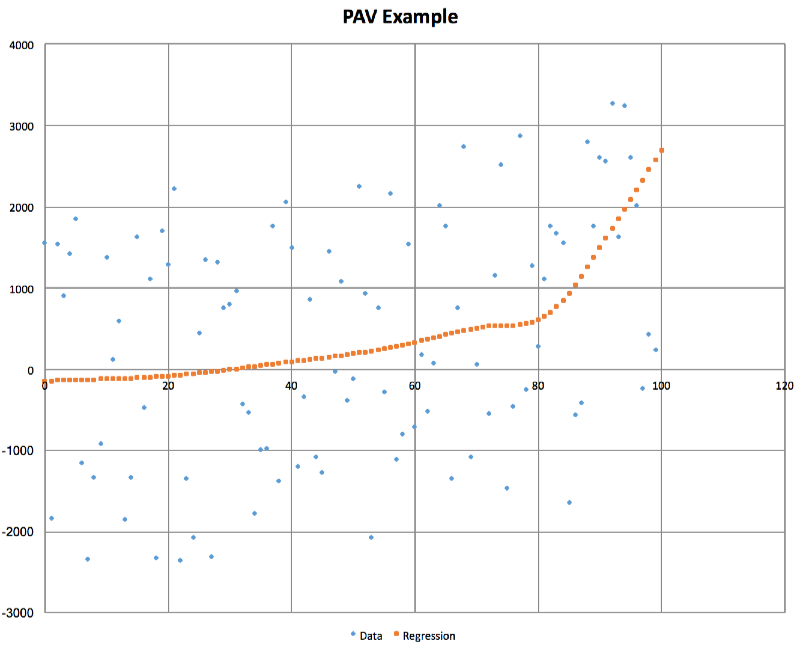Popularity
2.9
Stable
Activity
0.0
Stable
48
2
2

Programming language: Kotlin
Tags: Data Science

# pairAdjacentViolators alternatives and similar libraries

Based on the "Data Science" category.
Alternatively, view pairAdjacentViolators alternatives based on common mentions on social networks and blogs.

• ### optaplanner

Java Constraint Solver to solve vehicle routing, employee rostering, task assignment, maintenance scheduling, conference scheduling and other planning problems.
• ### kotlin-jupyter

Kotlin kernel for Jupyter/IPython
• ### Appwrite - The Open Source Firebase alternative introduces iOS support

Appwrite is an open source backend server that helps you build native iOS applications much faster with realtime APIs for authentication, databases, files storage, cloud functions and much more!
Promo
• ### kotlin-statistics

Idiomatic statistical operators for Kotlin
• ### Komputation

A neural network framework written in Kotlin.
• ### koma

A scientific computing library for Kotlin. https://kyonifer.github.io/koma
• ### KotlinDiscreteMathToolkit

Set of extensions for Kotlin that provides Discrete math functionalities

Do you think we are missing an alternative of pairAdjacentViolators or a related project?

### Popular Comparisons## Overview

An implementation of the Pair Adjacent Violators algorithm for isotonic regression. Written in Kotlin but usable from Java or any other JVM language.

Note this algorithm is also known as "Pool Adjacent Violators".

### What is "Isotonic Regression" and why should I care?

Imagine you have two variables, x and y, and you don't know the relationship between them, but you know that if x increases then y will increase, and if x decreases then y will decrease. Alternatively it may be the opposite, if x increases then y decreases, and if x decreases then y increases.

Examples of such isotonic or monotonic relationships include:

• x is the pressure applied to the accelerator in a car, y is the acceleration of the car (acceleration increases as more pressure is applied)
• x is the rate at which a web server is receiving HTTP requests, y is the CPU usage of the web server (server CPU usage will increase as the request rate increases)
• x is the price of an item, and y is the probability that someone will buy it (this would be a decreasing relationship, as x increases y decreases)

These are all examples of an isotonic relationship between two variables, where the relationship is likely to be more complex than linear.

So we know the relationship between x and y is isotonic, and let's also say that we've been able to collect data about actual x and y values that occur in practice.

What we'd really like to be able to do is estimate, for any given x, what y will be, or alternatively for any given y, what x would be required.

But of course real-world data is noisy, and is unlikely to be strictly isotonic, so we want something that allows us to feed in this raw noisy data, figure out the actual relationship between x and y, and then use this to allow us to predict y given x, or to predict what value of x will give us a particular value of y. This is the purpose of the pair-adjacent-violators algorithm.

#### ...and why should I care?

Using the examples I provide above:

• A self-driving car could use it to learn how much pressure to apply to the accelerator to give a desired amount of acceleration
• An autoscaling system could use it to help predict how many web servers they need to handle a given amount of web traffic
• A retailer could use it to choose a price for an item that maximizes their profit (aka "yield optimization")

#### Isotonic regression in online advertising

If you have an hour to spare, and are interested in learning more about how online advertising works - you should check out this lecture that I gave in 2015 where I explain how we were able to use pair adjacent violators to solve some fun problems.

#### A picture is worth a thousand words

Here is the relationship that PAV extracts from some very noisy input data where there is an increasing relationship between x and y:## Features

• Tries to do one thing and do it well with minimal bloat, no external dependencies (other than Kotlin's stdlib)
• Very thorough unit tests, achieving approx 75% mutation test coverage
• Employs an isotonic spline algorithm for smooth interpolation
• Fairly efficient implementation without compromizing code readability
• While implemented in Kotlin, works nicely from Java and other JVM languages
• Supports reverse-interpolation
• Will intelligently extrapolate to compute y for values of x greater or less than those used to build the PAV model

## Usage

You can use this library by adding a dependency for Gradle, Maven, SBT, Leiningen or another Maven-compatible dependency management system thanks to Jitpack:### Basic usage from Kotlin

``````import com.github.sanity.pav.PairAdjacentViolators
// ...
val inputPoints = listOf(Point(3.0, 1.0), Point(4.0, 2.0), Point(5.0, 3.0), Point(8.0, 4.0))
val interpolator = pav.interpolator()
println("Interpolated: \${interpolator(6.0)}")
``````

### Basic usage from Java

``````import com.github.sanity.pav.*;
import kotlin.jvm.functions.*;
import java.util.*;

public class PAVTest {
public static void main(String[] args) {
final Function1<Double, Double> interpolator = pav.interpolator();
for (double x=0; x<3; x+=0.1) {
System.out.println(x+"\t"+interpolator.invoke(x));
}
}
}
``````

### Questions/Feedback

Please ask questions, report bugs, request features etc via Github Issues, you're also more than welcome to submit pull requests.